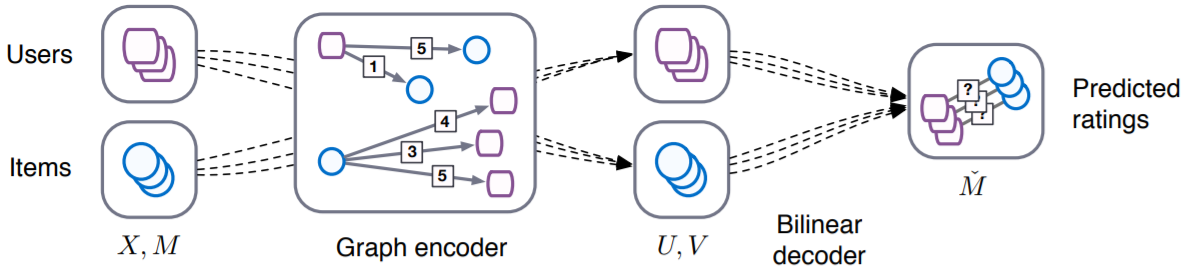GCMC¶

Introduction¶

[paper]

Title: Graph Convolutional Matrix Completion

Authors: Rianne van den Berg, Thomas N. Kipf, Max Welling

Abstract: We consider matrix completion for recommender systems from the point of view of link prediction on graphs. Interaction data such as movie ratings can be represented by a bipartite user-item graph with labeled edges denoting observed ratings. Building on recent progress in deep learning on graph-structured data, we propose a graph auto-encoder framework based on differentiable message passing on the bipartite interaction graph. Our model shows competitive performance on standard collaborative filtering benchmarks. In settings where complimentary feature information or structured data such as a social network is available, our framework outperforms recent state-of-the-art methods.Running with RecBole¶

Model Hyper-Parameters:

• accum (str) : The accumulation function in the GCN layers. Defaults to 'stack'. Range in ['sum', 'stack'].

• dropout_prob (float) : The dropout rate. Defaults to 0.3.

• gcn_output_dim (int) : The output dimension of GCN layer in GCN encoder. Defaults to 500.

• embedding_size (int) : The embedding size of user and item. Defaults to 64.

• sparse_feature (bool) : Whether to use sparse tensor to represent the features. Defaults to True.

• class_num (int) : Number of rating types. Defaults to 2.

• num_basis_functions (int) : Number of basis functions for BiDecoder. Defaults to 2.

A Running Example:

Write the following code to a python file, such as run.py

from recbole.quick_start import run_recbole

run_recbole(model='GCMC', dataset='ml-100k')

And then:

python run.py

Tuning Hyper Parameters¶

If you want to use HyperTuning to tune hyper parameters of this model, you can copy the following settings and name it as hyper.test.

learning_rate choice [0.01,0.005,0.001,0.0005,0.0001]
dropout_prob choice [0.0,0.1,0.2,0.3,0.4,0.5]
accum choice ['stack','sum']
gcn_output_dim choice [500,256,1024]
num_basis_functions choice ['2']

Note that we just provide these hyper parameter ranges for reference only, and we can not guarantee that they are the optimal range of this model.

Then, with the source code of RecBole (you can download it from GitHub), you can run the run_hyper.py to tuning:

python run_hyper.py --model=[model_name] --dataset=[dataset_name] --config_files=[config_files_path] --params_file=hyper.test

For more details about Parameter Tuning, refer to Parameter Tuning.

If you want to change parameters, dataset or evaluation settings, take a look at